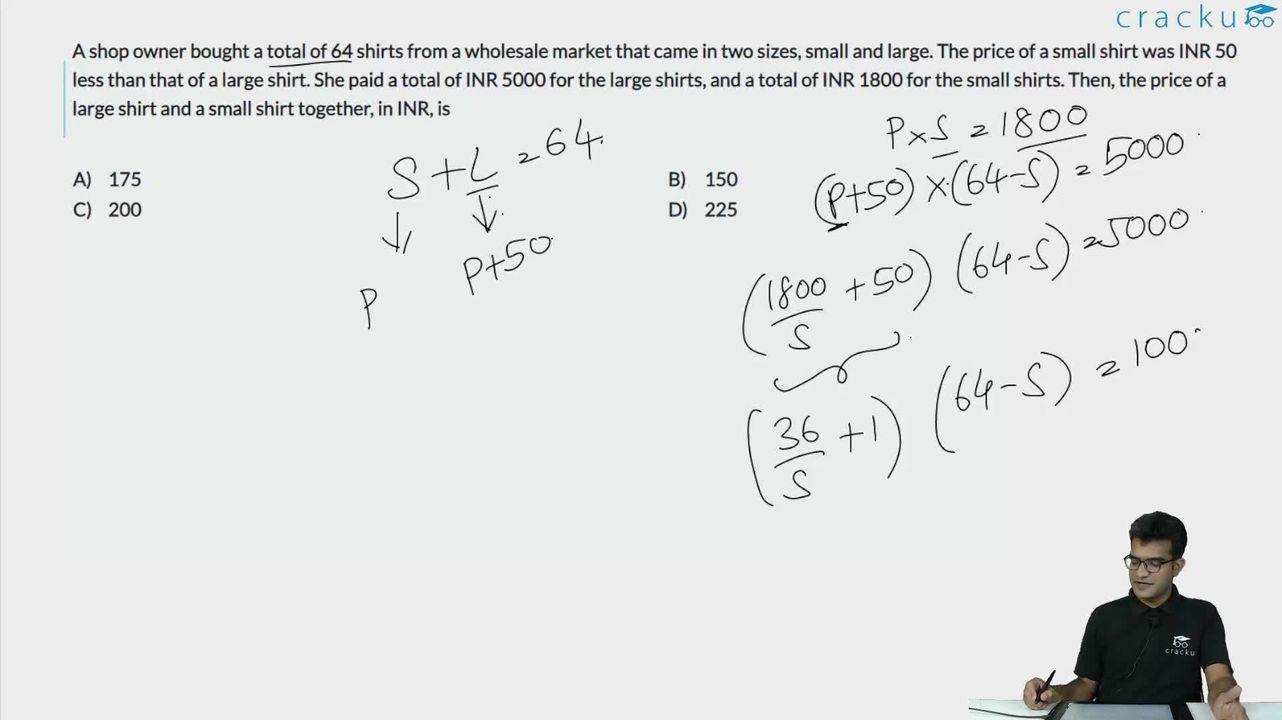Question 45

# A shop owner bought a total of 64 shirts from a wholesale market that came in two sizes, small and large. The price of a small shirt was INR 50 less than that of a large shirt. She paid a total of INR 5000 for the large shirts, and a total of INR 1800 for the small shirts. Then, the price of a large shirt and a small shirt together, in INR, is

Solution

Let the number of large shirts be l and the number of small shirts be s.

Let the price of a small shirt be x and that of a large shirt be x + 50.

Now, s + l = 64

l (x+50) = 5000

sx = 1800

lx + sx + 50l = 6800

64x +50l = 6800

Substituting l = (6800 - 64x) / 50, in the original equation, we get

$$\frac{\left(6800-64x\right)}{50}\left(x+50\right)=5000$$

(6800 - 64x)(x + 50) = 250000

$$6800x+340000-64x^2-3200x = 250000$$

$$64x^2-3600x-90000=0$$

Solving, we get, x= $$\frac{225\pm\ 375}{8}=\frac{600}{8}or-\frac{150}{8}$$

SO, x = 75

x + 50 = 125

Answer = 75 + 125 = 200.

Alternate approach: By options.

Hint: Each option gives the sum of the costs of one large and one small shirt. We know that large = small + 50

Hence, small + small + 50 = option.

SMALL = (Option - 50)/2

LARGE = Small + 50 = (Option + 50)/2

Option A and Option D gives us decimal values for SMALL and LARGE, hence we will consider them later.

Large = 150 + 50 / 2 = 100

Small = 150 - 50 / 2 = 50

Now, total shirts = 5000/100 + 1800/50 = 50 + 36 = 86 (X - This is wrong)

Option C -

Large = 200 + 50 / 2 = 125

Small = 200 - 50 / 2 = 75

Total shirts = 5000/125 + 1800/75 = 40 + 24 = 64 ( This is the right answer)

### View Video Solution